Mathematics: CUET Mock Test - 8

# Mathematics: CUET Mock Test - 8

Test Description

## 40 Questions MCQ Test CUET Science Subjects Mock Tests | Mathematics: CUET Mock Test - 8

Mathematics: CUET Mock Test - 8 for Class 12 2023 is part of CUET Science Subjects Mock Tests preparation. The Mathematics: CUET Mock Test - 8 questions and answers have been prepared according to the Class 12 exam syllabus.The Mathematics: CUET Mock Test - 8 MCQs are made for Class 12 2023 Exam. Find important definitions, questions, notes, meanings, examples, exercises, MCQs and online tests for Mathematics: CUET Mock Test - 8 below.
Solutions of Mathematics: CUET Mock Test - 8 questions in English are available as part of our CUET Science Subjects Mock Tests for Class 12 & Mathematics: CUET Mock Test - 8 solutions in Hindi for CUET Science Subjects Mock Tests course. Download more important topics, notes, lectures and mock test series for Class 12 Exam by signing up for free. Attempt Mathematics: CUET Mock Test - 8 | 40 questions in 45 minutes | Mock test for Class 12 preparation | Free important questions MCQ to study CUET Science Subjects Mock Tests for Class 12 Exam | Download free PDF with solutions
 1 Crore+ students have signed up on EduRev. Have you?
Mathematics: CUET Mock Test - 8 - Question 1

### If f(x) =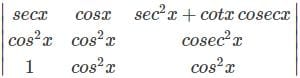then what is the value of 0∫π/2 f(x) dx = (π/4 + 8/15)?

Detailed Solution for Mathematics: CUET Mock Test - 8 - Question 1

(dy/dx) = (dx/dy)-1
So, d2y/dx2 = -(dx/dy)-2 d/dx(dx/dy)
= -(dy/dx)2(d2x/dy2)(dy/dx)
= d2y/dx2 + (dy/dx)3 d2y/dx2 = 0

Mathematics: CUET Mock Test - 8 - Question 2

### What will be the value of x if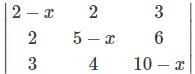= 0?

Detailed Solution for Mathematics: CUET Mock Test - 8 - Question 2

Here, we have,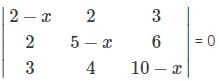Now, replacing C3 = C3 – 3C1, we get,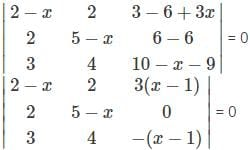Or, (x – 1)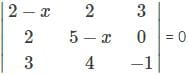Now, replacing R1 = R1 + 3R3, we get,
Or, (x – 1)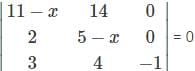Or, (x – 1)[-1 {(11 – x)(5 – x) – 28}] = 0
Or, -(x – 1)(55 – 11x – 5x + x2 – 28)
Or, (x – 1)(x2 – 16x + 27) = 0
Thus, either x – 1 = 0 i.e. x = 1 or x2 – 16x + 27 = 0
Therefore, solving x2 – 16x + 27 = 0 further, we get,
x = 8 ± √37

Mathematics: CUET Mock Test - 8 - Question 3

### Find the area of the triangle with the vertices (2,3), (4,1), (5,0).

Detailed Solution for Mathematics: CUET Mock Test - 8 - Question 3

The area of the triangle with vertices (2,3), (4,1), (5,0) is given by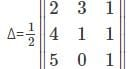Applying R2→R2-R3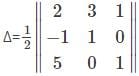Expanding along R2, we get
Δ=(1/2){-(-1)(3-0)+1(2-5)}
Δ=(1/2) (0-0)=0.

Mathematics: CUET Mock Test - 8 - Question 4

For which of the elements in the determinant Δ=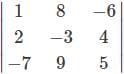the cofactor is -37.

Detailed Solution for Mathematics: CUET Mock Test - 8 - Question 4

Consider the element -3 in Δ=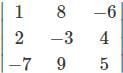The cofactor of the element -3 is given by
A22=(-1)2+2 M22
M22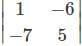=1(5)-(-6)(-7)=5-42=-37
A22=(-1)2+2 (-37)=-37.

Mathematics: CUET Mock Test - 8 - Question 5

Find the determinant of the matrix A=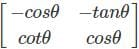.

Detailed Solution for Mathematics: CUET Mock Test - 8 - Question 5

Given that, A=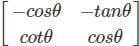|A| =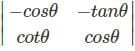|A|=-cos⁡θ (cos⁡θ )-cotθ(-tan⁡θ)
|A|=-cos2⁡θ+1=sin2⁡θ.

Mathematics: CUET Mock Test - 8 - Question 6

What will be the value of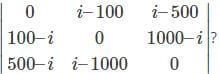Detailed Solution for Mathematics: CUET Mock Test - 8 - Question 6

The above matrix is a skew symmetric matrix and its order is odd
And we know that for any skew symmetric matrix with odd order has determinant = 0
Therefore, the value of the given determinant = 0

Mathematics: CUET Mock Test - 8 - Question 7

What is the relation between the two determinants f(x) =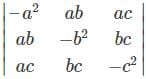and g(x) =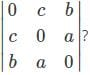Detailed Solution for Mathematics: CUET Mock Test - 8 - Question 7

Let, D =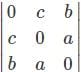Expanding D by the 1st row we get,
D = – c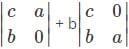= – c(0 – ab) + b(ac – 0)
= 2abc
Now, we have adjoint of D = D’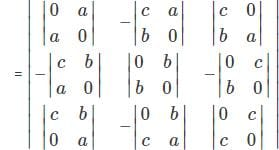Or, D’ =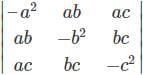Or, D’ = D2
Or, D’ = D2 = (2abc)2

Mathematics: CUET Mock Test - 8 - Question 8

Find the equation of the line joining A(5,1), B(4,0) using determinants.

Detailed Solution for Mathematics: CUET Mock Test - 8 - Question 8

Let C(x,y) be a point on the line AB. Thus, the points A(5,1), B(4,0), C(x,y) are collinear. Hence, the area of the triangle formed by these points will be 0.
⇒ Δ = (1/2)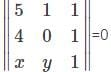Applying R1→R1-R2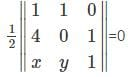Expanding along R1, we get
=(1/2) {1(0-y)-1(4-x)}=0
=(1/2){-y-4+x}=0
⇒ x-y = 4.

Mathematics: CUET Mock Test - 8 - Question 9

For which of the following elements in the determinant Δ=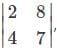the minor of the element is 2?

Detailed Solution for Mathematics: CUET Mock Test - 8 - Question 9

Consider the element 7 in the determinant Δ=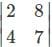The minor of the element 7 can be obtained by deleting R2 and C2
∴ M22 = 2
Hence, the minor of the element 7 is 2.

Mathematics: CUET Mock Test - 8 - Question 10

Evaluate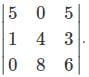Detailed Solution for Mathematics: CUET Mock Test - 8 - Question 10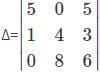Expanding along R1, we get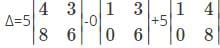Δ=5(24-24)-0+5(8-0)
Δ=0-0+40=40.

Mathematics: CUET Mock Test - 8 - Question 11

If f(x) =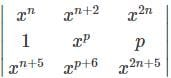= 0,then what will be the value of p?

Detailed Solution for Mathematics: CUET Mock Test - 8 - Question 11

Here, C1 and C3 becomes equal when we put p = xn
And R1 and R3 becomes equal when we put p = n + 1
And R1 and R3 becomes equal when we put p = n + 1

Mathematics: CUET Mock Test - 8 - Question 12

Find the value of k for which the points (3, 2), (1, 2), (5, k) are collinear.

Detailed Solution for Mathematics: CUET Mock Test - 8 - Question 12

Given that the vertices are (3,2), (1,2), (5,k)
Therefore, the area of the triangle with vertices (3,2), (1,2), (5,k) is given by
Δ=(1/2)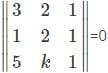Applying R1→R1-R2, we get
1/2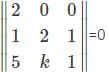Expanding along R1, we get
(1/2) {2(2-k)-0+0} = 0
2-k = 0
k = 2 .

Mathematics: CUET Mock Test - 8 - Question 13

For which of the following element in the determinant Δ=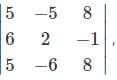the minor and the cofactor both are zero.

Detailed Solution for Mathematics: CUET Mock Test - 8 - Question 13

Consider the element 2 in the determinant Δ=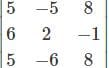The minor of the element 2 is given by
∴ M22 =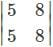= 40-40 = 0
⇒ A22 = (-1)2+2 (0) = 0.

Mathematics: CUET Mock Test - 8 - Question 14

Which of the following conditions holds true for a system of equations to be consistent?

Detailed Solution for Mathematics: CUET Mock Test - 8 - Question 14

If a given system of equations has one or more solutions then the system is said to be consistent.

Mathematics: CUET Mock Test - 8 - Question 15

Differentiate (log⁡2x)sin⁡3x with respect to x.

Detailed Solution for Mathematics: CUET Mock Test - 8 - Question 15

Consider y=(log2x)sin3x
Applying log on both sides, we get
log⁡y=log(log2x)sin3x
log⁡y=sin⁡3x log⁡(log⁡2x)
Differentiating with respect to x, we get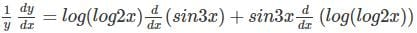By using chain rule, we get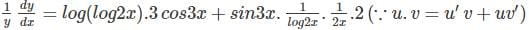dy/dx =y(3 cos⁡3x log⁡(log⁡2x)+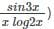∴ dy/dx=log⁡2xsin⁡3x(3cos3xlog(log2x)+(sin3x/xlog2x))

Mathematics: CUET Mock Test - 8 - Question 16

Find the second order derivative of y=9 log⁡ t3.

Detailed Solution for Mathematics: CUET Mock Test - 8 - Question 16

Given that, y=9 log⁡t3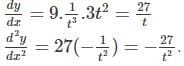Mathematics: CUET Mock Test - 8 - Question 17

Differentiate 8e-x+2ex w.r.t x.

Detailed Solution for Mathematics: CUET Mock Test - 8 - Question 17

To solve:y=(8e-x+2ex)
Differentiating w.r.t x we get,
(dy/dx)= 8(-e-x+2ex)
∴ (dy/dx)= 2ex - 8e-x.

Mathematics: CUET Mock Test - 8 - Question 18

If the rate of change of radius of a circle is 6 cm/s then find the rate of change of area of the circle when r=2 cm.

Detailed Solution for Mathematics: CUET Mock Test - 8 - Question 18

The rate of change of radius of the circle is dr/dt = 6 cm/s
The area of a circle is A=πr2
Differentiating w.r.t t we get,
(dA/dt = d/dt) (πr2) = 2πr (dr/dt) =2πr(6)=12πr.
dA/dt |r=2=24π= 24×3.14=75.36 cm2/s

Mathematics: CUET Mock Test - 8 - Question 19

A given systems of equations is said to be inconsistent if _____

Detailed Solution for Mathematics: CUET Mock Test - 8 - Question 19

If a given system of equations has no solutions, then the system is said to be inconsistent.

Mathematics: CUET Mock Test - 8 - Question 20

Differentiate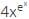with respect to x.

Detailed Solution for Mathematics: CUET Mock Test - 8 - Question 20

Consider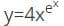Applying log on both sides, we get
log⁡y=log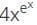log⁡y=log⁡4+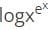(∵log⁡ab  =log⁡a+log⁡b)
Differentiating both sides with respect to x, we get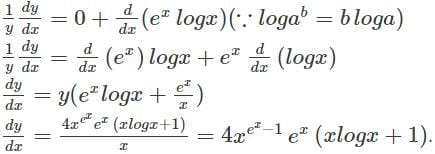Mathematics: CUET Mock Test - 8 - Question 21

Find d2y/dx2, if y=tan2⁡x+3 tan⁡x.

Detailed Solution for Mathematics: CUET Mock Test - 8 - Question 21

Given that, y=tan2⁡⁡x+3 tan⁡x
dy/dx =2 tan⁡x sec2⁡⁡x+3 sec2⁡x=sec2⁡⁡x (2 tan⁡x+3)
By using the u.v rule, we get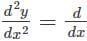(sec2⁡⁡x).(2 tan⁡x+3)+ (d/dx) (2 tan⁡x+3).sec2⁡⁡x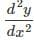=2 sec2⁡⁡x tan⁡x (2 tan⁡x+3)+sec2⁡⁡x (2 sec⁡x tanx)
=2 sec2⁡x tan⁡x (2 tan⁡x+sec⁡x+3).

Mathematics: CUET Mock Test - 8 - Question 22

Differentiate 8ecos2x w.r.t x.

Detailed Solution for Mathematics: CUET Mock Test - 8 - Question 22

Consider y=8ecos2x
Differentiating w.r.t x by using chain rule, we get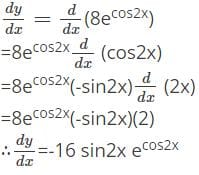Mathematics: CUET Mock Test - 8 - Question 23

The edge of a cube is increasing at a rate of 7 cm/s. Find the rate of change of area of the cube when x=6 cm.

Detailed Solution for Mathematics: CUET Mock Test - 8 - Question 23

Let the edge of the cube be x. The rate of change of edge of the cube is given by dx/dt =7cm/s.
The area of the cube is A=6x2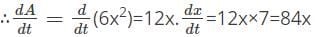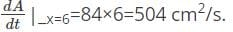Mathematics: CUET Mock Test - 8 - Question 24

Find the value of x and y for the given system of equations.
3x+2y=6
5x+y=2

Detailed Solution for Mathematics: CUET Mock Test - 8 - Question 24

By using the matrix method, the given equations can be expressed in the form of the equation AX=B, where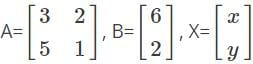To find the value of x and y, we need to solve the matrix X
X = A-1 B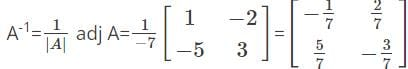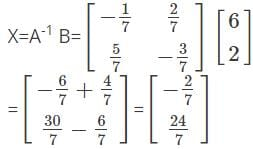Mathematics: CUET Mock Test - 8 - Question 25

Differentiate 9tan⁡3x with respect to x.

Detailed Solution for Mathematics: CUET Mock Test - 8 - Question 25

Consider y=9tan⁡3x
Applying log on both sides, we get
log⁡y=log⁡9tan⁡3x
Differentiating both sides with respect to x, we get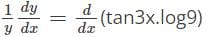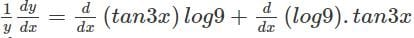(∵ Using u.v = u′v + uv′)
(dy/dx) = y(3 sec2⁡⁡x.log⁡9+0)
(dy/dx) = 9tan⁡3x (3 log⁡9 sec2⁡x)

Mathematics: CUET Mock Test - 8 - Question 26

Differentiate 3 sin-1⁡(e2x) w.r.t x.

Detailed Solution for Mathematics: CUET Mock Test - 8 - Question 26

Consider y=3 sin-1⁡(e2x)
dy/dx = d/dx (3 sin-1⁡(e2x))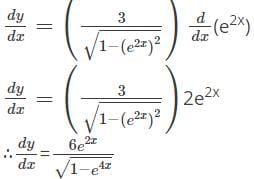Mathematics: CUET Mock Test - 8 - Question 27

The rate of change of area of a square is 40 cm2/s. What will be the rate of change of side if the side is 10 cm.

Detailed Solution for Mathematics: CUET Mock Test - 8 - Question 27

Let the side of the square be x.
A = x2, where A is the area of the square
Given that,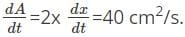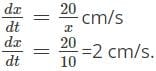Mathematics: CUET Mock Test - 8 - Question 28

Differentiate (cos⁡3x)3x with respect to x.

Detailed Solution for Mathematics: CUET Mock Test - 8 - Question 28

Consider y=(cos⁡3x)3x
Applying log on both sides, we get
log⁡y=log⁡(cos⁡3x)3x
log⁡y=3x log⁡(cos⁡3x)
Differentiating both sides with respect to x, we get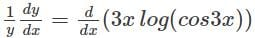By using u.v=u’ v+uv’, we get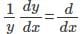(3x)log(cos3x)+(d/dx)(log(cos3x)).3x
(dy/dx)=y(3 log⁡(cos⁡3x) +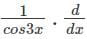(cos3x).3x)
(dy/dx)=y(3 log⁡(cos⁡3x) +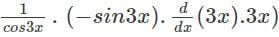(dy/dx)=y(3 log⁡(cos⁡3x) +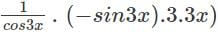(dy/dx) = y(3 log⁡(cos⁡3x) – 9x tan⁡3x)
(dy/dx) = (cos⁡3x)3x (3 log⁡(cos⁡3x) – 9x tan⁡3x)

Mathematics: CUET Mock Test - 8 - Question 29

Find the second order derivative of y=2e2x-3 log⁡(2x-3).

Detailed Solution for Mathematics: CUET Mock Test - 8 - Question 29

Given that, y=2e2x-3 log⁡(2x-3)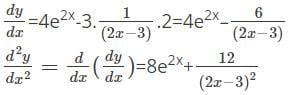Mathematics: CUET Mock Test - 8 - Question 30

Differentiate log⁡(log⁡x5) w.r.t x.

Detailed Solution for Mathematics: CUET Mock Test - 8 - Question 30

Consider y=(log⁡(log⁡(x5)))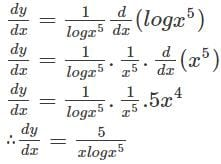Mathematics: CUET Mock Test - 8 - Question 31

The total cost P(x) in rupees associated with a product is given by P(x)=0.4x2+2x-10. Find the marginal cost if the no. of units produced is 5.

Detailed Solution for Mathematics: CUET Mock Test - 8 - Question 31

The Marginal cost is the rate of change of revenue w.r.t the no. of units produced, we get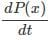= 0.8x+2
cost(MC)=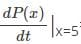=0.8x+2=0.8(5)+2=4+2=6.

Mathematics: CUET Mock Test - 8 - Question 32

Find the value of x, y, z for the given system of equations.
2x+3y+2z=50
x+4y+3z=40
3x+3y+5z=60

Detailed Solution for Mathematics: CUET Mock Test - 8 - Question 32

The given system of equations can be expressed in the form of AX=B, where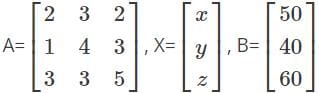X = A-1 B
∴ A-1 = (1/|A|) adj A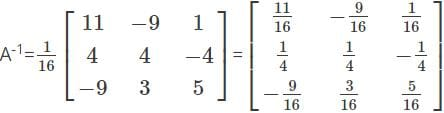X = A-1 B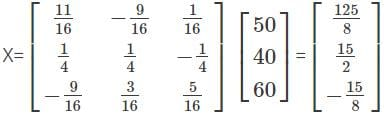Mathematics: CUET Mock Test - 8 - Question 33

Differentiate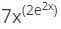with respect to x.

Detailed Solution for Mathematics: CUET Mock Test - 8 - Question 33

Consider y=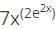log⁡y=log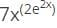log⁡y=log⁡7+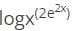log⁡y=log⁡7+2e2x log⁡x
Differentiating with respect to x on both sides, we get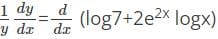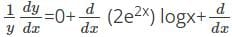(log⁡x)2e2x (using u.v=u’ v+uv’)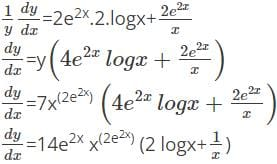Mathematics: CUET Mock Test - 8 - Question 34

Find d2y/dx2, if y=2 sin-1⁡(cos⁡x).

Detailed Solution for Mathematics: CUET Mock Test - 8 - Question 34

Given that, y=2 sin-1⁡(cos⁡x)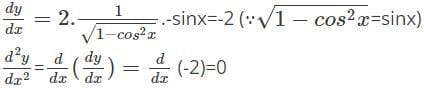Mathematics: CUET Mock Test - 8 - Question 35

Differentiate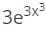w.r.t x.

Detailed Solution for Mathematics: CUET Mock Test - 8 - Question 35

Consider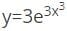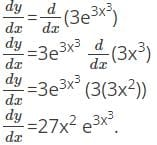Mathematics: CUET Mock Test - 8 - Question 36

At what rate will the lateral surface area of the cylinder increase if the radius is increasing at the rate of 2 cm/s when the radius is 5 cm and height is 10 cm?

Detailed Solution for Mathematics: CUET Mock Test - 8 - Question 36

Let r be the radius and h be the height of the cylinder. Then, dr/dt =2 cm/s
The area of the cylinder is given by A=2πrh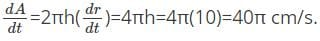Mathematics: CUET Mock Test - 8 - Question 37

Differentiate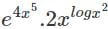with respect to x.

Detailed Solution for Mathematics: CUET Mock Test - 8 - Question 37

Consider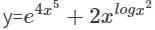Applying log on both sides, we get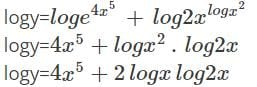Differentiating with respect to x, we get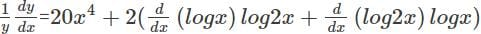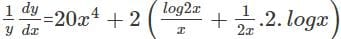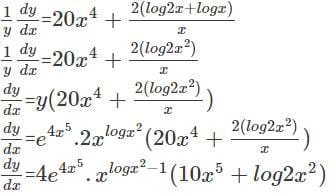Mathematics: CUET Mock Test - 8 - Question 38

If y=log⁡(2x3), find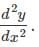Detailed Solution for Mathematics: CUET Mock Test - 8 - Question 38

Given that, y=log⁡(2x3)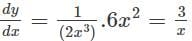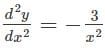Mathematics: CUET Mock Test - 8 - Question 39

Differentiate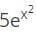tan⁡x w.r.t x.

Detailed Solution for Mathematics: CUET Mock Test - 8 - Question 39

Consider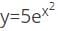tan⁡x
Differentiating w.r.t x by using chain rule, we get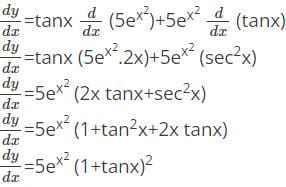Mathematics: CUET Mock Test - 8 - Question 40

If the circumference of the circle is changing at the rate of 5 cm/s then what will be rate of change of area of the circle if the radius is 6cm.

Detailed Solution for Mathematics: CUET Mock Test - 8 - Question 40

The circumference of the circle is given by C=2πr, where r is the radius of the circle.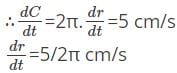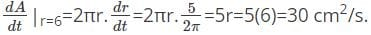## CUET Science Subjects Mock Tests

60 tests
 Use Code STAYHOME200 and get INR 200 additional OFF Use Coupon Code
Information about Mathematics: CUET Mock Test - 8 Page
In this test you can find the Exam questions for Mathematics: CUET Mock Test - 8 solved & explained in the simplest way possible. Besides giving Questions and answers for Mathematics: CUET Mock Test - 8, EduRev gives you an ample number of Online tests for practice

60 tests